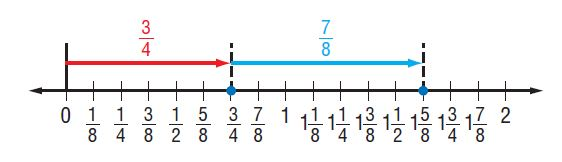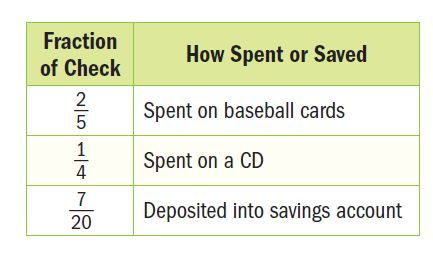Homework Explained - Math Practice 101Dear guest, you are not a registered member. As a guest, you only have read-only access to our books, tests and other practice materials.

As a registered member you can:

Registration is free and doesn't require any type of payment information. Click here to Register.
Go to page:
Chapter 4: Rational Numbers; Chapter Review• Question 1

Jeremy ran a mile in 5 minutes and 8 seconds. Write the time in minutes as a decimal.

•  $$min$$
• Question 2

Amaya received a $$\large\frac{26}{30}$$ on her English test and an 81% on her biology test. On which test did she receive the higher score? Explain.

•  $$\text{English test}$$ $$\text{biology test}$$
• Question 3

Model with Mathematics Mrs. Brown made two desserts. The number line below shows the number of cups of sugar she used to make them. Write an addition sentence that shows the amount of sugar Mrs. Brown used.• Question 4

Lucas babysat his younger sister $$\large2\frac{1}{2}$$ hours on Friday and $$\large3\frac{2}{3}$$ hours on Saturday. How much longer did Lucas babysit his younger sister on Saturday than on Friday?

• $$\text{h}$$
• Question 5

Financial Literacy For his birthday, Aiden received a check from his grandmother. The table shows how he spent or saved the money. Two weeks later, Aiden withdrew $$\large\frac{2}{3}$$ of the money he had deposited into his savings account. What fraction in simplest form of the original check did Aiden withdraw? Explain.• Question 6

The world’s largest bird is the ostrich, whose mass can be as much as 156.5 kilograms. What is the approximate weight in pounds?

•  $$\text{ lb }$$
• Question 7

An ounce is $$\frac{1}{16}$$ of a pound. How many ounces are in $$\large8\frac{3}{4}$$ pounds?

•  $$\text{oz}$$

Yes, email page to my online tutor. (if you didn't add a tutor yet, you can add one here)